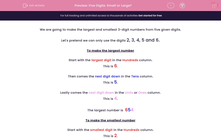# Five Digits: Small or Large?

In this worksheet, students are given five digits from which they must identify the smallest and largest three-digit numbers.Key stage:  KS 2

Curriculum topic:   Maths and Numerical Reasoning

Curriculum subtopic:   Place Value

Difficulty level:#### Worksheet Overview

We are going to make the largest and smallest 3-digit numbers from five given digits.

Let's pretend we can only use the digits 2, 3, 4, 5 and 6.

To make the largest number

This is 6.

Then comes the next digit down in the Tens column.

This is 5.

Lastly comes the next digit down in the Units or Ones column.

This is 4.

The largest number is  654

To make the smallest number

This is 2.

Then comes the next largest digit in the Tens column.

This is 3.

Lastly comes the next largest digit  in the Units or Ones column.

This is 4.

The largest number is  234

Example

Using any 3 of the digits from:

68671

form the smallest and largest 3-digit numbers that you can make.

Put the digits in order:

16678

1 is the smallest digit

6 is the next largest digit.

6 is the next largest digit even though it is the same as the last one.

Smallest number is 166.

8 is the largest digit

7 is the next digit down.

6 is the next digit down.

Largest number is 876.

### What is EdPlace?

We're your National Curriculum aligned online education content provider helping each child succeed in English, maths and science from year 1 to GCSE. With an EdPlace account you’ll be able to track and measure progress, helping each child achieve their best. We build confidence and attainment by personalising each child’s learning at a level that suits them.

Get started••••